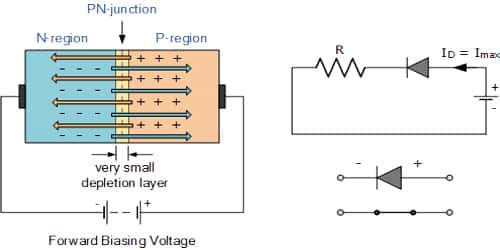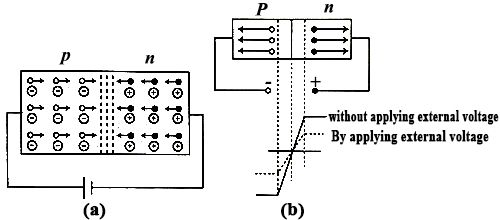Physics

# Forward bias Working principle of Junction DiodeForward bias Working principle of Junction Diode

If a p-type and n-type semiconductors are connected by a special technique then the junction surface is called a p-n junction. It is one of the simplest semiconductor devices around, and which has the characteristic of passing current in only one direction only. When a voltage is applied across a diode in such a way that the diode allows current, the diode is said to be forward-biased. From external potential difference can be applied in the p-n junction in two ways, viz— forward bias and reverse bias. Here describe forward bias working principle.

(a) Forward bias: When the external voltage in the junction is applied in such a way that by reducing the potential barrier current flows, then it means that forward bias has been applied. In case of applying forward, bias in the junction positive terminal of the battery is connected to the end of the p-type region and the negative terminal is connected to the end of the n-type region. In figure (a) and (b) forward biasing and a decrease of the potential barrier due to forward biasing have been shown. Applied potential difference creates an electric field which acts in opposition to the electric field of the potential barrier. So, the resultant field decreases and the height or width of the barrier decreases. Since the voltage of the potential bather is very small (0.1 to 0.3 V); so, for small applied forward-voltage width of the barrier decreases. As a result junction resistance is removed and current starts flowing. Since positive terminal of the battery is connected to the p-type material so positive, the terminal of the battery will attract the electrons toward the left i.e., towards p-type material and negative terminal being connected to the n-type region; so negative terminal will attract holes towards right i.e., towards the n-type material. Now electrons are repelled by the ‘n-terminal’ and from the n-type material move to the p-type material by crossing the junction.Similarly, positive holes being repelled by the positive end of the battery move to the ‘n-material’ crossing the junction. At the junction holes and electrons combine. Again the negative terminal of the battery supplies fresh electrons to the n-type semiconductor and positive end of the battery attracting electrons from the p-type semiconductor creates new holes. As a result, continuous charge, i.e., current flows. The value of this current is of the order of mA. This current is called ‘forward current’.